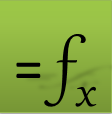## Optimizing cone radius and height for a specified volume

The volume of cone of base radius R and height H is given by

${V}_{cone}=\frac{\pi {R}^{2}H}{3}$

It can be computed numerically by the following triple integral in Cartesian coordinates:

${V}_{cone}=\underset{-R}{\overset{R}{\int }}dx\underset{-\sqrt{{R}^{2}-{x}^{2}}}{\overset{\sqrt{{R}^{2}-{x}^{2}}}{\int }}dy\underset{\frac{H}{R}\sqrt{{x}^{2}+{y}^{2}}}{\overset{H}{\int }}dz$

In this excercise we will compute the required Height or Radius for a specified volume based on the triple integral with dynamical optimizaion.

### What is the required height to obtain a cone volume of 1.5?

Using X1, Y1, Z1 as the integration variables, we define formulas for the triple integral limits in D5:D7 as shown in Table 2. We name the limits formulas respectively as z_low, y_low, and y_hi. We also define two variables H and Rd (using B1 and B2) to serve as our design parameters with initial values of 1 as shown in Table 1. The exact volume is computed in B3. To compute the triple integral, we nest QUADF for the inner, middle and outer integrals in B5, B6 and B7 passing in the proper integration variable and limits for each integral as shown in Table 1. Note that the integrand for inner integral in this example is a constant one, therefore it is unnecessary to define a formula for the integrand and we pass the number 1 directly in the first argument to QUADF in B5. The inner integral B5 formula serves as the integrand for the middle integral B6 which in turn, serves as as the integrand for the outer integral B7. Evaluating B7 computes the triple integral volume accurately as shown in Table 1.

 A B B 1 H 1 2 Rd 1 3 Exact Vol =PI()*Rd^2*H/3 1.047197551 5 I_dz =QUADF(1,Z1,z_low,H) 6 I_dy =QUADF(B5,Y1,y_low,y_hi) 7 Vol =QUADF(B6,X1,-Rd,Rd) 1.047197541
 C D 5 z_low =H/Rd*SQRT(X1^2+Y1^2) 6 y_low =-SQRT(Rd^2-X1^2) 7 y_hi =SQRT(Rd^2-X1^2)

To find H that will produce a volume of 1.5, we define the constraint =B7-1.5 in B10 which penalizes the difference between the computed cone volume in B7 and the target value of 1.5. Next, we use NLSOLVE to solve for H that will drive this constraint to zero. We evaluate the formula =NLSOLVE(B10,H) in B11 which computes the answer as shown Table 3. Using the computed value for H, we verify the volume in B12 which is 1.5 as expected.

 A B B 10 constraint =B7-1.5 11 H =NLSOLVE(B10,H) 1.432394502 12 Actual vol =PI()*R^2*B11/3 1.500000015

The computed value of H is quite accurate thanks to the default adaptive algorithm for QUADF. However, we can also cut computational time by changing the algorithm for the outer integration in B7 to a fixed-point QK15 algorithm. We modify B7 formula as follows =QUADF(B6,X1,-Rd,Rd,{"Algor","QK15"}). The gain in speed comes at a slight loss of accuracy (1.499 versus 1.500) as shown in Table 4.

 A B 11 H 1.431621252 12 Actual vol 1.499190269
Question or Comment? Email us:
support @ excel-works.comExceLab: Transforming Excel into a Calculus Power House

ExceLab functions and methods are protected by USA Patents 10628634, 10114812, 9892108 and 9286286.